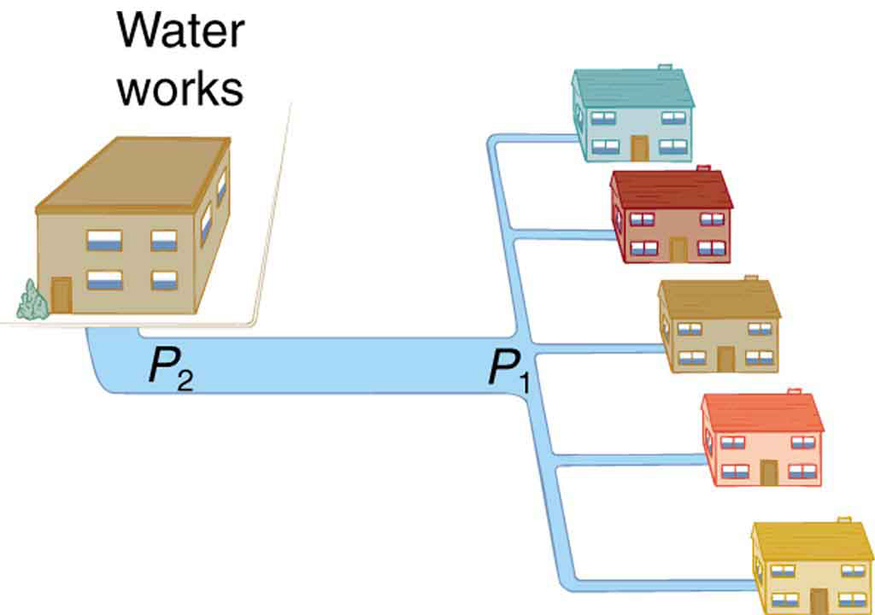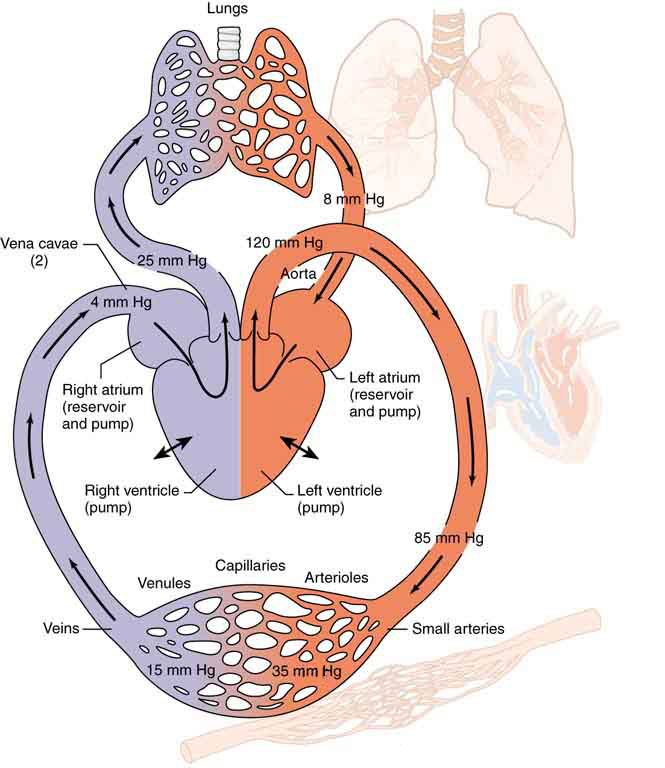# 12.4 Viscosity and laminar flow; poiseuille’s law  (Page 5/12)

 Page 5 / 12
$Q=\frac{{P}_{2}-{P}_{1}}{R}$

to

${P}_{2}-{P}_{1}=RQ\text{,}$

where, in this case, ${P}_{2}$ is the pressure at the water works and $R$ is the resistance of the water main. During times of heavy use, the flow rate $Q$ is large. This means that ${P}_{2}-{P}_{1}$ must also be large. Thus ${P}_{1}$ must decrease. It is correct to think of flow and resistance as causing the pressure to drop from ${P}_{2}$ to ${P}_{1}$ . ${P}_{2}-{P}_{1}=RQ$ is valid for both laminar and turbulent flows.During times of heavy use, there is a significant pressure drop in a water main, and P 1 supplied to users is significantly less than P 2 created at the water works. If the flow is very small, then the pressure drop is negligible, and P 2 ≈ P 1 size 12{P rSub { size 8{2} } approx P rSub { size 8{1} } } {} .

We can use ${P}_{2}-{P}_{1}=RQ$ to analyze pressure drops occurring in more complex systems in which the tube radius is not the same everywhere. Resistance will be much greater in narrow places, such as an obstructed coronary artery. For a given flow rate $Q$ , the pressure drop will be greatest where the tube is most narrow. This is how water faucets control flow. Additionally, $R$ is greatly increased by turbulence, and a constriction that creates turbulence greatly reduces the pressure downstream. Plaque in an artery reduces pressure and hence flow, both by its resistance and by the turbulence it creates.

[link] is a schematic of the human circulatory system, showing average blood pressures in its major parts for an adult at rest. Pressure created by the heart’s two pumps, the right and left ventricles, is reduced by the resistance of the blood vessels as the blood flows through them. The left ventricle increases arterial blood pressure that drives the flow of blood through all parts of the body except the lungs. The right ventricle receives the lower pressure blood from two major veins and pumps it through the lungs for gas exchange with atmospheric gases – the disposal of carbon dioxide from the blood and the replenishment of oxygen. Only one major organ is shown schematically, with typical branching of arteries to ever smaller vessels, the smallest of which are the capillaries, and rejoining of small veins into larger ones. Similar branching takes place in a variety of organs in the body, and the circulatory system has considerable flexibility in flow regulation to these organs by the dilation and constriction of the arteries leading to them and the capillaries within them. The sensitivity of flow to tube radius makes this flexibility possible over a large range of flow rates.Schematic of the circulatory system. Pressure difference is created by the two pumps in the heart and is reduced by resistance in the vessels. Branching of vessels into capillaries allows blood to reach individual cells and exchange substances, such as oxygen and waste products, with them. The system has an impressive ability to regulate flow to individual organs, accomplished largely by varying vessel diameters.

Each branching of larger vessels into smaller vessels increases the total cross-sectional area of the tubes through which the blood flows. For example, an artery with a cross section of $1\phantom{\rule{0.25em}{0ex}}{\text{cm}}^{2}$ may branch into 20 smaller arteries, each with cross sections of $0.5\phantom{\rule{0.25em}{0ex}}{\text{cm}}^{2}$ , with a total of $\text{10}\phantom{\rule{0.25em}{0ex}}{\text{cm}}^{2}$ . In that manner, the resistance of the branchings is reduced so that pressure is not entirely lost. Moreover, because $Q=A\overline{v}$ and $A$ increases through branching, the average velocity of the blood in the smaller vessels is reduced. The blood velocity in the aorta ( $\text{diameter}=1\phantom{\rule{0.25em}{0ex}}\text{cm}$ ) is about 25 cm/s, while in the capillaries ( $\text{20}\mu \text{m}$ in diameter) the velocity is about 1 mm/s. This reduced velocity allows the blood to exchange substances with the cells in the capillaries and alveoli in particular.

topic-- question
Salman
I know this is unrelated to physics, but how do I get the MCQs and essay to work. they arent clickable.
20cm3 of 1mol/dm3 solution of a monobasic acid HA and 20cm3 of 1mol/dm3 solution of NaOH are mixed in a calorimeter and a temperature rise of 274K is observed. If the heat capacity of the calorimeter is 160J/K, calculate the enthalpy of neutralization of the acid.(SHCw=4.2J/g/K) Formula. (ms*cs+C)*T
why is a body moving at a constant speed able to accelerate
20cm3 of 1mol/dm3 solution of a monobasic acid HA and 20cm3 of 1mol/dm3 solution of NaOH are mixed in a calorimeter and a temperature rise of 274K is observed. If the heat capacity of the calorimeter is 160J/K, calculate the enthalpy of neutralization of the acid.(SHCw=4.2J/g/K) Formula. (ms*cs+C)*T
Lilian
because it changes only direction and the speed is kept constant
Justice
Why is the sky blue...?
It's filtered light from the 2 forms of radiation emitted from the sun. It's mainly filtered UV rays. There's a theory titled Scatter Theory that covers this topic
Mike
A heating coil of resistance 30π is connected to a 240v supply for 5min to boil a quantity of water in a vessel of heat capacity 200jk. If the initial temperature of water is 20°c and it specific heat capacity is 4200jkgk calculate the mass of water in a vessel
A thin equi convex lens is placed on a horizontal plane mirror and a pin held 20 cm vertically above the lens concise in position with its own image the space between the undersurface of d lens and the mirror is filled with water (refractive index =1•33)and then to concise with d image d pin has to
Be raised until its distance from d lens is 27cm find d radius of curvature
Azummiri
what happens when a nuclear bomb and atom bomb bomb explode add the same time near each other
A monkey throws a coconut straight upwards from a coconut tree with a velocity of 10 ms-1. The coconut tree is 30 m high. Calculate the maximum height of the coconut from the top of the coconut tree? Can someone answer my question
v2 =u2 - 2gh 02 =10x10 - 2x9.8xh h = 100 ÷ 19.6 answer = 30 - h.
Ramonyai
why is the north side is always referring to n side of magnetic
who is a nurse
A nurse is a person who takes care of the sick
Bukola
a nurse is also like an assistant to the doctor
explain me wheatstone bridge
good app
samuel
Wheatstone bridge is an instrument used to measure an unknown electrical resistance by balancing two legs of a bridge circuit, one leg of which includes the unknown component.
MUHD
Rockwell Software is Rockwell Automation’s "Retro Encabulator". Now, basically the only new principle involved is that instead of power being generated by the relative motion of conductors and fluxes, it’s produced by the modial interaction of magneto-reluctance and capacitive diractance. The origin
Chip
what refractive index
write a comprehensive note on primary colours
relationship between refractive index, angle of minimum deviation and angle of prism
Harrison
Who knows the formula for binding energy,and what each variable or notation stands for?ByByBy Anonymous UserByByBy